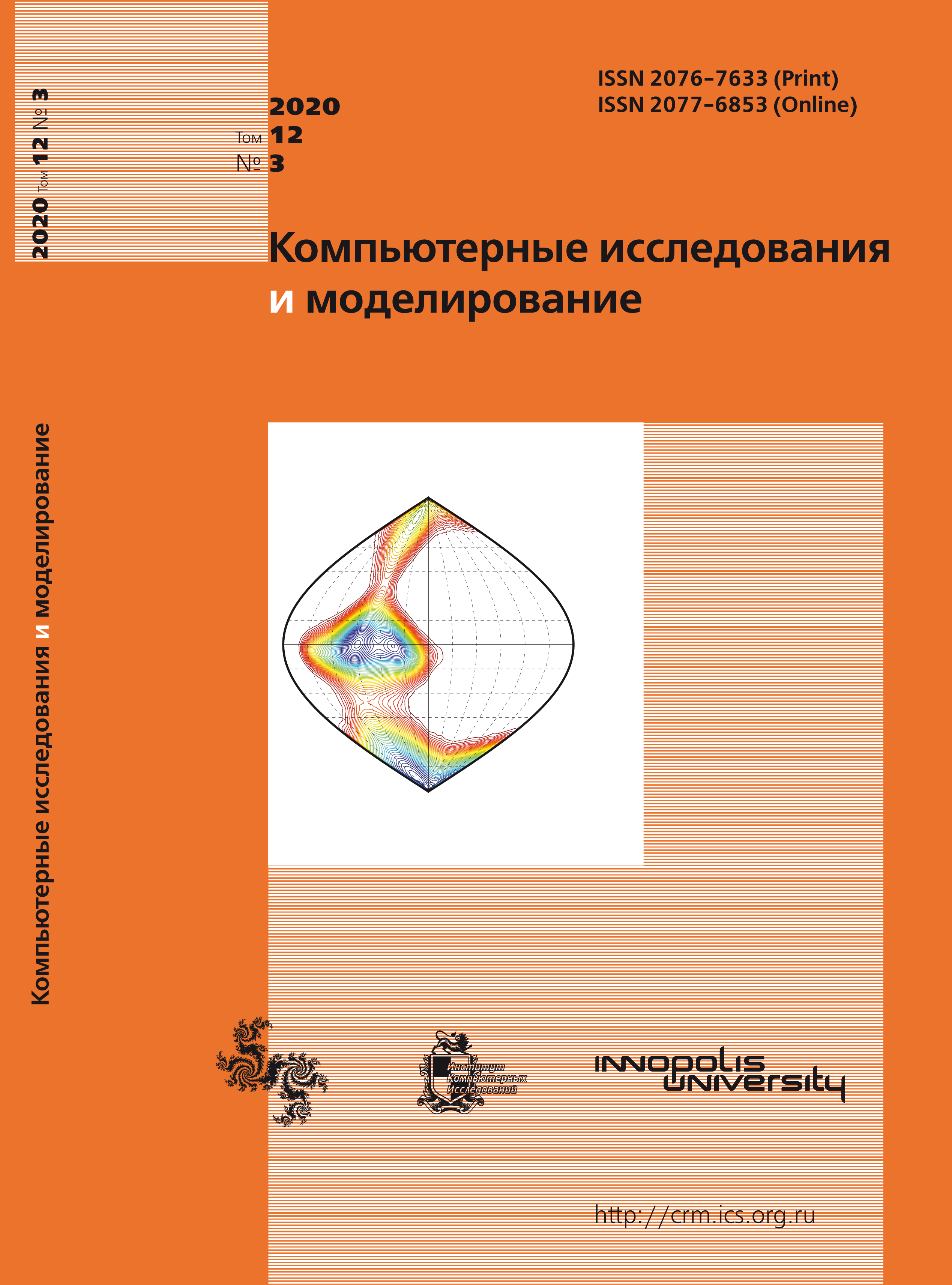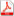Issue 3, 2020 Vol. 12

# All issues

Effect of buoyancy force on mixed convection of a variable density fluid in a square lid-driven cavitypdf (762K)

The paper considers the problem of stationary mixed convection and heat transfer of a viscous heatconducting fluid in a plane square lid-driven cavity. The hot top cover of the cavity has any temperature $T_\mathrm{H}$ and cold bottom wall has temperature $T_\mathrm{0} (T_\mathrm{H} > T_\mathrm{0})$, whereas in contrast the side walls are insulated. The fact that the fluid density can take arbitrary values depending on the amount of overheating of the cavity cover is a feature of the problem. The mathematical formulation includes the Navier–Stokes equations in the ’velocity–pressure’ variables and the heat balance equation which take into account the incompressibility of the fluid flow and the influence of volumetric buoyancy force. The difference approximation of the original differential equations has been performed by the control volume method. Numerical solutions of the problem have been obtained on the $501 \times 501$ grid for the following values of similarity parameters: Prandtl number Pr = 0.70; Reynolds number Re = 100 and 1000; Richardson number Ri = 0.1, 1, and 10; and the relative cover overheating $(T_\mathrm{H}-T_\mathrm{0})/T_\mathrm{0} = 0, 1, 2, 3$. Detailed flow patterns in the form of streamlines and isotherms of relative overheating of the fluid flow are given in the work. It is shown that the increase in the value of the Richardson number (the increase in the influence of buoyancy force) leads to a fundamental change in the structure of the liquid stream. It is also found out that taking into account the variability of the liquid density leads to weakening of the influence of Ri growth on the transformation of the flow structure. The change in density in a closed volume is the cause of this weakening, since it always leads to the existence of zones with negative buoyancy in the presence of a volumetric force. As a consequence, the competition of positive and negative volumetric forces leads in general to weakening of the buoyancy effect. The behaviors of heat exchange coefficient (Nusselt number) and coefficient of friction along the bottom wall of the cavity depending on the parameters of the problem are also analyzed. It is revealed that the greater the values of the Richardson number are, the greater, ceteris paribus, the influence of density variation on these coefficients is.

Keywords: Navier–Stokes equations, viscous fluid, arbitrary change in density, heat transfer, mixed convection, lid-driven cavity, numerical simulation
Citation in English: Fomin A.A., Fomina L.N. Effect of buoyancy force on mixed convection of a variable density fluid in a square lid-driven cavity // Computer Research and Modeling, 2020, vol. 12, no. 3, pp. 575-595
Citation in English: Fomin A.A., Fomina L.N. Effect of buoyancy force on mixed convection of a variable density fluid in a square lid-driven cavity // Computer Research and Modeling, 2020, vol. 12, no. 3, pp. 575-595
DOI: 10.20537/2076-7633-2020-12-3-575-595

Full-text version of the journal is also available on the web site of the scientific electronic library eLIBRARY.RU

The journal is included in the Russian Science Citation Index

The journal is included in the List of Russian peer-reviewed journals publishing the main research results of PhD and doctoral dissertations.

International Interdisciplinary Conference "Mathematics. Computing. Education"

The journal is included in the RSCI

Indexed in Scopus